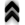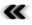### 6.5 Geometric Structures

As explored in Section 4.6, curves (Deﬁnition 45) are a versatile entity in the context of diﬀerential geometry as well as in physical applications as seen in Section 5.3. Geometric structures such as curves are deﬁned on top of topological spaces, whose discrete representation has already been outlined in Section 6.3. The structure of ﬁber bundles as described in Section 6.4 allows for a simple storage of vector ﬁelds (Deﬁnition 62), which are intrinsically connected to their integral curves (Deﬁnition 63). This connected notion should be carried over from the continuous setting to the world of digital computers.

However, since the data items, such as vector ﬁelds, are stored only on discrete points, associated with the CW complex (Deﬁnition 34), a curve can also only be approximated. Returning to a very basic notion of the curve, as a mapping (Deﬁnition 20) associating a single parameter to a set of points in a manifold (Deﬁnition 37), it becomes apparent that an approximation of a curve can be achieved by storing a set of points with an additional mapping relating the CW complex and the curve.

Thus an integral curve, as a one-dimensional diﬀerentiable manifold, has its own representation, which is initially completely independent of the topological space it traverses. However, a mapping must exist and also be explicitly available, in order to use a curve within the discrete digital world. This mapping is readily found, when turning to atlases (Deﬁnition 36) and their charts. Thus by calculating the coordinates of points, it is possible to relate the points of the curve with its embedding manifold. However, in a manifold the obtained coordinates are valid only locally and worthless, since they degenerate to a mere collection of numbers, without knowledge of the chart in which they are obtained. A simple example is obtained by specifying points using barycentric coordinates within each of the cells. Without knowledge which cell to reference it is not possible to correctly locate the point in the CW complex. In the case of an aﬃne space (Deﬁnition 79), the obtained coordinates have global scope and no further information needs to be speciﬁed, beside the knowledge that it is indeed an aﬃne space.

The points comprising the curve can either be represented using a CW complex but can also be given in an implicit manner, using a prescription to determine points from a parameter. In the case of integral curves, the derivative with respect to the parameter of the mapping used for point calculations must match the given vector ﬁeld. The simple yet powerful structure of polynomials (Deﬁnition 19) makes them well suited for this task. In order to avoid issues with high polynomial orders due to Runge’s phenomenon  interpolation is usually restricted to locally using low orders of polynomials, thus modelling the curve piecewise by several polynomials. This piecewise representation of a curve has become known as a spline.

Since the local nature of splines corresponds to the local nature of charts, they are well suited to directly provide coordinates for points of the curve, making them directly applicable in the previously described scheme.

The identiﬁcation of points using coordinates seems to alleviate the problem of dealing with points belonging to two manifold structures, the solution suﬀers from imperfections due to the numerical issues connected with the calculation of the coordinates. It thus degrades the reliability of determining the identity of points, as it moves it from a problem related to set theory and topology to a geometric setting.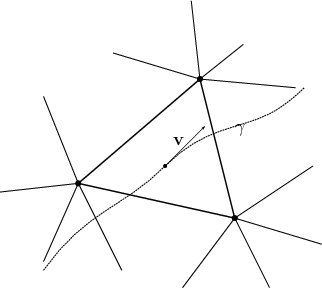Figure 6.2: A curve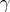passes through several elements, thus as the curve’s parameter is varied the location corresponds to diﬀerent cells.

In case the description uses local expressions, corresponding to cells, it is important to identify, when a curve moves from one cell to the next (illustrated in Figure 6.2), as it indicates the limit of its validity. Thus, the intersection of a curve with the boundary of a cell is worth to be investigated further.

In the case of a Riemannian base manifold and hence the availability of a mapping between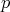-forms and vectors due to an isomorphism as indicated in Equation 4.156, the boundaries of tetrahedral and hypercube cells can be geometrically represented using a normal vector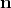to the boundaries surface using a simple formula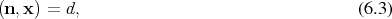where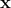represents a point to be tested.

Given the curve has a local polynomial representation in a parameter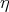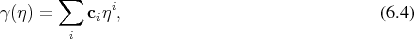where the coeﬃcients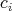are connected to the current cell, e.g., derived from quantities associated to this cell. Inserting Equation 6.4 into Equation 6.3, the intersections are obtainable as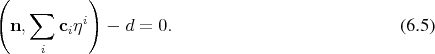Linearity allows to reformulate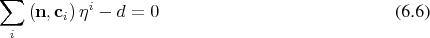showing the resulting polynomial in the parameter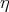, whose coeﬃcients are given by the inner product of the curve parameters and the normal vector, whose roots indicate the possible intersections. The correct intersection can then be chosen from the calculated roots.

As a machine does not possess any intuitive notion which boundary component may be intersected and which not, the intersection needs to be evaluated for every boundary component (sub-cell) of the currently investigated cell. The boundary sub-cells and their face normals thereby need to be available from the base topological framework.

The following piece of code illustrates an implementation of the outlined algorithm using the GSSE infrastructure.

Listing 6.1: Determination of points of transit from cell to cell. Data type deﬁnitions.
typedef gsse::boundary<DIM, DIMB, CellTopology> GFacetOnCell;
typedef gsse::result_of::boundary<DIM, DIMB, CellTopology>::type
GFacetOnCell_result;

gsse::container<3, numeric_t> ci;

These data types are deﬁned and evaluated at compile time, where DIM represents the global manifold dimension and DIMB represents the corresponding facet sub dimension. The type CellTopology describes the discrete cell representation of the underlying manifold as explicit mesh, e.g., a simplex mesh or a cube grid, as outlined is Section 6.3. Finally, the ci need to be available or calculated form values deﬁned on the base topology, as sketched in Section 6.4, to represent the curve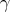according to Equation 6.4. Here a cubic polynomial, thus of degree, is chosen for illustration. An application of this procedure, where the coeﬃcients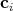are determined from physical considerations, is available in Section 7.2.1.

Where the previous snippet of code presented a compile time structure, the next piece of code is evaluated at run time. The new_cell along with the roots of the curve parameter are calculated from the given current cell, its boundaries and their geometry, and the ci as indicated by the global mesh structure.

Listing 6.2: Determination of points of transit from cell to cell. Run time calculation.
GFacetOnCell         facet_on_cell;
GFacetOnCell_result  facet_result = facet_on_cell(cell);
Cell new_cell;

for (size_t i = 0; i < gsse::size(facet_result); ++i)
{
gsse::vec_t nvec = gsse::normal_vector(facet_result [i]);
numeric_t   d    = gsse::in(nvec, facet_result[i] );

gsse::polynomial<3,numeric_t>   poly(0, d);
gsse::algorithm::traverse (poly,
gsse::in( nvec, gsse::acc(ci)) );
gsse::vec_t roots = gsse::poly_root_calculator( poly );

if (roots_valid (roots) )
{ new_cell = gsse::set::not_in (
cell_on_facet( facet_result[i]) , cell
);
break
}
}

The local description due to the use of splines limits the order of the used polynomials, thereby also limiting complications connected to the calculation of the roots of the polynomial. Once the next cell has been determined, the next polynomial of the spline corresponding to the new cell can be used.